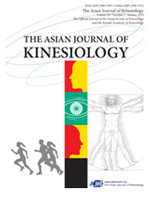상세검색
최근 검색어 전체 삭제
다국어입력
즐겨찾기0KCI등재 학술저널

# Correlation and Reliability of Two Field Tests for Vertical Jump Height

• 등재여부 : KCI등재
• 2020.01
• 9 - 14 (6 pages)

OBJECTIVES This study established the relationship and reliability of vertical jump height measurements using both the jump-reach method and the flight-time method. METHODS Sixteen healthy subjects (13 males and 3 females, body mass index: 22.5 kg/m2) visited the laboratory twice with at least two days between visits. During each visit, they performed three successful trials of one- and two-legged maximal vertical jumps on a Vertec jump tester (the jump-reach method). Simultaneously, two digital cameras were videotaped, one for a whole body view and another for a view of the feet (240 frame rate and 1/1000 s shutter speed). Flight-times were measured using a free motion analysis software (Kinovea 0.8.15) and were then inserted into the formula h = t2 × 122.625 to calculate the height. To determine if the jump values from each method were correlated, average values from the three trials for both jump methods were analyzed using Pearson correlation and simple linear regression tests. To establish a within- and between-session reliability, the intraclass correlation coefficients (ICCs) were calculated. RESULTS Assessed vertical jump heights using the two methods were highly correlated with each other (r values ranged between 0.86 and 0.93 with p<0.0001 for all tests) and showed high reliability (ICC values ranged between 0.73 and 0.99 for all tests). CONCLUSIONS Vertical jump heights assessed by the two field tests were highly correlated and consistent. Although the flight-time method calculates less jump heights, the underestimated amount can be estimated by the established equations. We suggest that athletes and coaches use either technique in the field in consideration of advantages for each method.

Introduction

Methods

Results

Discussion

Conclusions

Conflicts of Interest

References

로딩중